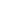Flat 50% off

Ends in

•• 11th
•• Chemistry
• Back to Doubt Clearing

## Without knowing the value of reaction quotient which of the following reaction can be predicated to go to completion at 25ºC?(a) CH$_3$OCH$_3$($l$) $\leftrightharpoons$ CH$_3$OCH$_3$($g$)(b) H$_2$O($l$) $\leftrightharpoons$ H$_2$O($g$)(c) H$_2$($g$) $\leftrightharpoons$ 2H($g$)(d) Sulphur ($s$) $\leftrightharpoons$ sulphar ($g$)

### Asked By syed shoaib hussaini

Updated Wed, 21 Nov 2018 03:15 pm